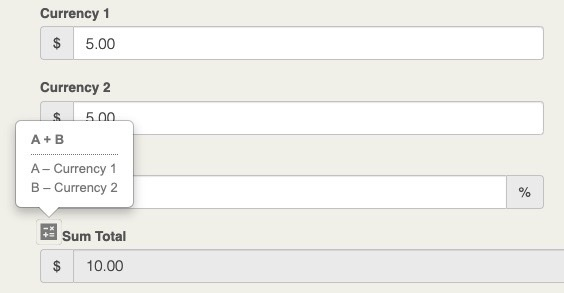Documents

# Calculated Fields

December 31, 2021

## Save Time and Prevent Human Error on Calculations

Filevine is taking automation up a notch with calculated fields that do the work for you. With four types of calculated fields—currency, decimal, number, and percentage—easily establish the equations you want while also determining formatting of the displayed outcome.

Using predefined formulas variable field types, calculated fields will display the results of the formula you set and will update in real time as any of the variable field amounts are changed. A useful calculator symbol next to the calculated fields will help confirm the calculated results with an explanation of the selected formula and variables.Calculated fields can be used in reports, DocGen, and in a project’s vitals, however, formulas are limited to using fields from within either the same project section or same collection section.